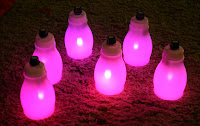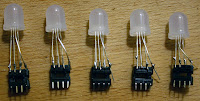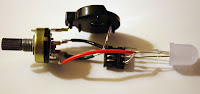## Monday, May 17, 2010

### Pixie-Dust BottlesIt occurred to me whilst making some RGB nightlights for the kids that it'd be fun if they could make the lights change to whatever colour they wanted; kinda like the Philips LivingColors lamp, but about \$100 cheaper. Lin suggested that having a few of these (say 5 - 7) would give the kids more room for imaginative fun, so I set about making a prototype and then a few more when that was up and working.

The kids love them (which is always good) and have been claiming that the bottles contain pixie dust (hence the title of this post).

Can you guess what Ffion's favourite colour is? She claims that this is the 'right colour' for pixie dust.The hardware is pretty straight forward. I used a potentiometer with an in-built on/off switch, like this one, to simplify things. I thought it'd look much nicer than having a separate switch. The rest of the parts are the same as for the RGB nightlights project. The trickiest part was the coding, mainly because I'm doing it late at night when my brain isn't working quite so well...

Ingredients:1. Potentiometer with switch
2. ATtiny45
3. 8-pin IC socket
4. coin cell battery holder
5. RGB LED (common anode) - I bought mine from EMSL. These are 10mm diffused RGB LEDs.
6. hookup wire, solder etc.

Methods:

I soldered the coin cell holder onto the potentiometer first.

Then I hooked up the RGB LED to the DIP socket. I'm using common anode LEDs so the long LED lead is soldered onto pin 8 of the IC socket. I have the RGB channels/leads connected to pins 5, 6 and 7 and the potentiometer output (middle pin from the pot) connected to pin 3.After that, all you have to do is solder on the potentiometer's middle terminal to the IC socket and finish off connecting the ground and power connections.And there we have it. Some kind of strange Borg eye ready to be put somewhere.

We had some plastic kids drink bottles sitting in the recycling bin - they're made from white plastic so I thought they'd diffuse the light nicely. I drilled a hole in the bottle cap for the potentiometer shaft and fitted it all together. Finally, I added a knob (radioshack purchase) to make it a bit prettier and easier to use.Coding:

I'd already worked out how to do the software PWM (pulse width modulation) and how to measure a variable voltage input using ADC (analogue to digital conversion) so all that needed to be figured out, for this project, was how to map the ADC input values to the desired range of colours.

I wanted to let the kids cycle through the entire spectrum in much the same way the previous night-lights cycled automatically.

So, in the RGB nightlights project there were 6 transitions/steps:
stepRed channel valueGreen channel valueBlue channel valuechanging channelcolour range
125500increase GreenRed to Yellow
22552550decrease RedYellow to Green
302550increase BlueGreen to Cyan
40255255decrease GreenCyan to Blue
500255increase RedBlue to Purple
62550255decrease BluePurple to Red

If we use a 10-bit ADC we have 1024 values (2^10) available for mapping to colours in the above steps. This means that each step can contain 170 values/colours (1024/6 = 170.666...).

So, to map the ADC value to a colour we first assign it to one of the 6 steps and then use it to determine the value of the varying channel (Red/Green/Blue) for that step.

There are 6 steps and each step can have 170 ADC values associated with it. So I binned the ADC values into steps like so:
1< 170
2< 342
3< 512
4< 683
5< 854
6>= 854

Here's an example to clarify: Say the potentiometer is set just over half way, then the ADC value should be between 512 and 683; this would put us in step 4 where the red channel is off, the blue channel is completely on (255) and the green channel is varying (in this step the green levels are decreasing from 255 -> 0 as the ADC value increases from 512 -> 683). We calculate the green level by translating the ADC range to 0 - 170 (in this case by subtracting 512) and then multiplying by a scaling factor (255/170) to transform the 0 -> 170 range to a 0 -> 255 range. If we're increasing the level of the channel (steps 1, 3 and 5) then we just use this scaled value as the channel value. If we're decreasing the level of the channel (as is the case for step 4) then we inverse the scaled value by subtracting it from 255.

Here's the bit of code that sets the RGB values (see the complete listing here: selectableColourLight.c):

```#define SCALING_RANGE 170
#define SCALING_FACTOR 255/SCALING_RANGE

...

void setRgbLevels(uint16_t pValue)
{
if(pValue < SCALING_RANGE)
{
mRgbValues[RED_INDEX]   = 255;
mRgbValues[GREEN_INDEX] = pValue * SCALING_FACTOR;
mRgbValues[BLUE_INDEX]  = 0;

}
else if(pValue < 342) //SCALING_RANGE * 2
{
mRgbValues[RED_INDEX]   = 255 - ((pValue - SCALING_RANGE) * SCALING_FACTOR);
mRgbValues[GREEN_INDEX] = 255;
mRgbValues[BLUE_INDEX]  = 0;
}
else if(pValue < 512) //SCALING_RANGE * 3
{
mRgbValues[RED_INDEX]   = 0;
mRgbValues[GREEN_INDEX] = 255;
mRgbValues[BLUE_INDEX]  = (pValue - 342) * SCALING_FACTOR;
}
else if(pValue < 683)//SCALING_RANGE * 4
{
mRgbValues[RED_INDEX]   = 0;
mRgbValues[GREEN_INDEX] = 255 - ((pValue - 512) * SCALING_FACTOR);
mRgbValues[BLUE_INDEX]  = 255;
}
else if(pValue < 854)//SCALING_RANGE * 5
{
mRgbValues[RED_INDEX]   = (pValue - 683) * SCALING_FACTOR;
mRgbValues[GREEN_INDEX] = 0;
mRgbValues[BLUE_INDEX]  = 255;
}
else
{
mRgbValues[RED_INDEX]   = 255;
mRgbValues[GREEN_INDEX] = 0;
mRgbValues[BLUE_INDEX]  = 255 - ((pValue - 854) * SCALING_FACTOR);
}
}```